Type
Quiz
Book Title
Fundamentals of Corporate Finance Standard Edition 9th Edition
ISBN 13
978-0073382395

### 978-0073382395 Chapter 11 Questions and Problems 19-22

April 3, 2019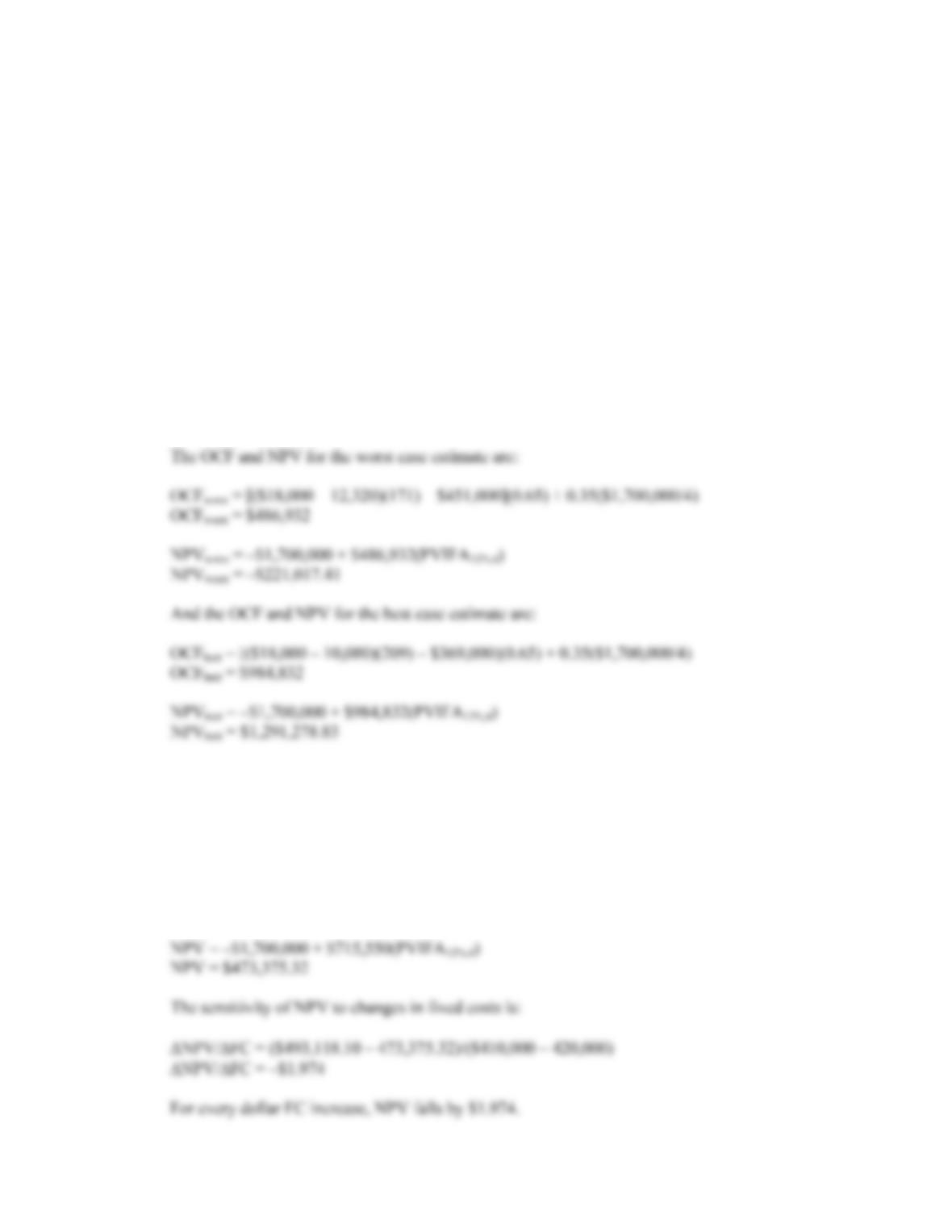CHAPTER 11 B-211
19. a. The base-case, best-case, and worst-case values are shown below. Remember that in the best-case,
sales and price increase, while costs decrease. In the worst-case, sales and price decrease, and costs
increase.
Scenario Unit sales Variable cost Fixed costs
Base 190 \$11,200 \$410,000
Best 209 \$10,080 \$369,000
Worst 171 \$12,320 \$451,000
Using the tax shield approach, the OCF and NPV for the base case estimate is:
OCFbase = [(\$18,000 – 11,200)(190) – \$410,000](0.65) + 0.35(\$1,700,000/4)
OCFbase = \$722,050
NPVbase = –\$1,700,000 + \$722,050(PVIFA12%,4)
NPVbase = \$493,118.10
b. To calculate the sensitivity of the NPV to changes in fixed costs we choose another level of fixed
costs. We will use fixed costs of \$420,000. The OCF using this level of fixed costs and the other
base case values with the tax shield approach, we get:
OCF = [(\$18,000 – 11,200)(190) – \$410,000](0.65) + 0.35(\$1,700,000/4)
OCF = \$715,550
And the NPV is: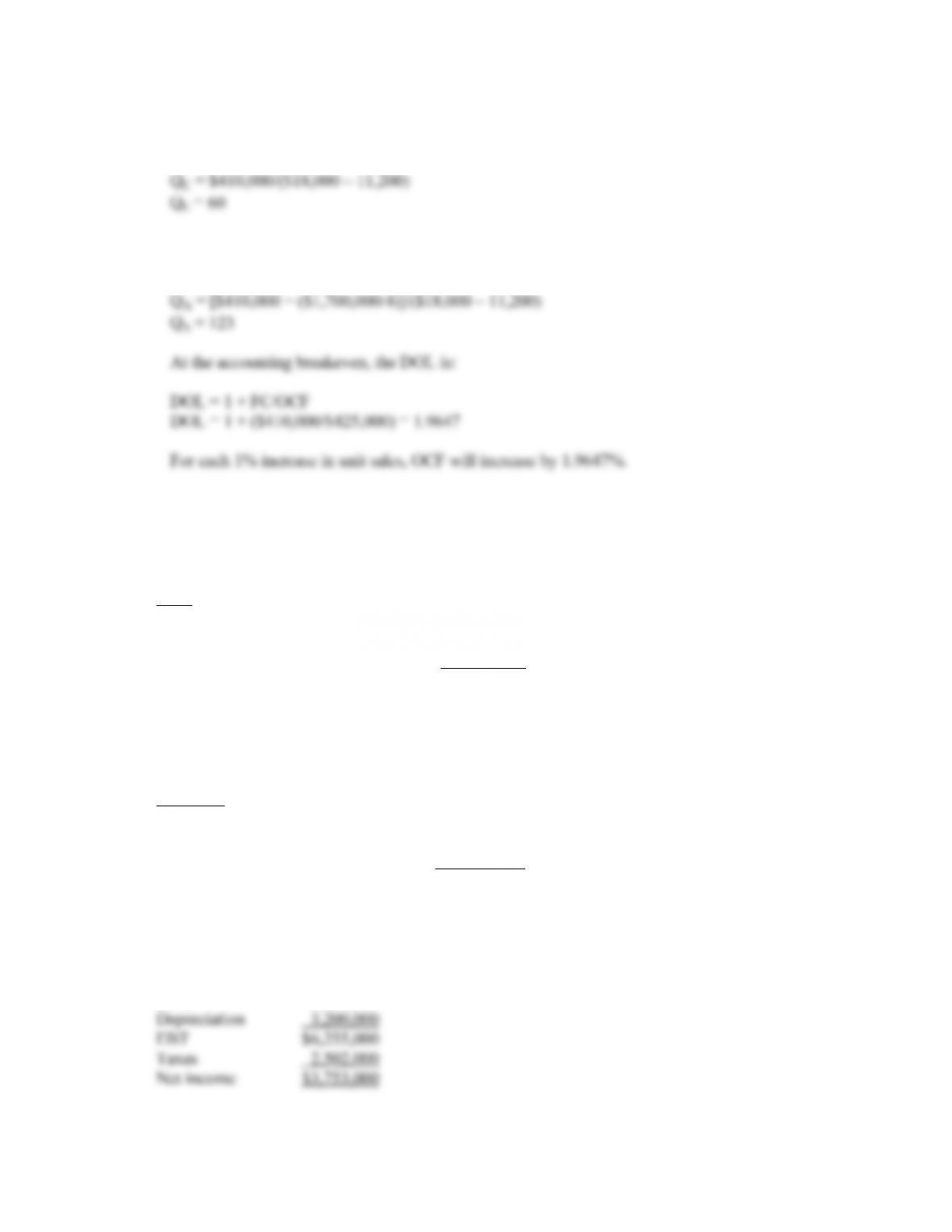B-212 SOLUTIONS
c. The cash breakeven is:
Q
C = FC/(P – v)
Q
d. The accounting breakeven is:
Q
A = (FC + D)/(P – v)
Q
20. The marketing study and the research and development are both sunk costs and should be ignored. We
will calculate the sales and variable costs first. Since we will lose sales of the expensive clubs and gain
sales of the cheap clubs, these must be accounted for as erosion. The total sales for the new project will
be:
Sales
New clubs \$750 51,000 = \$38,250,000
Exp. clubs \$1,200 (–11,000) = –13,200,000
Cheap clubs \$420 9,500 = 3,990,000
\$29,040,000
For the variable costs, we must include the units gained or lost from the existing clubs. Note that the
variable costs of the expensive clubs are an inflow. If we are not producing the sets anymore, we will
save these variable costs, which is an inflow. So:
Var. costs
New clubs –\$330 51,000 = –\$16,830,000
Exp. clubs –\$650 (–11,000) = 7,150,000
Cheap clubs –\$190 9,500 = –1,805,000
–\$11,485,000
The pro forma income statement will be:
Sales \$29,040,000
Variable costs 11,485,000
Costs 8,100,000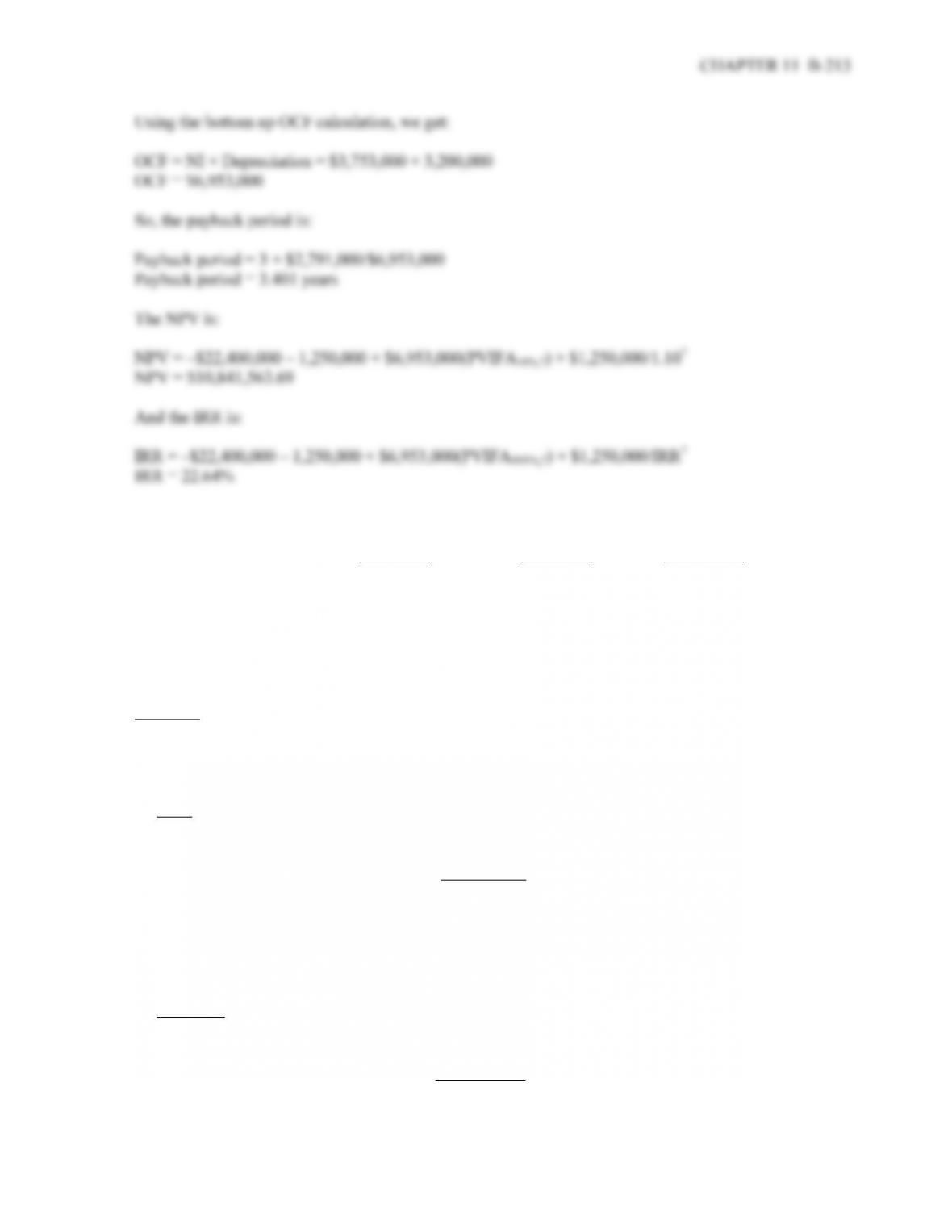21. The best case and worst cases for the variables are:
Base Case Best Case Worst Case
Unit sales (new) 51,000 56,100 45,900
Price (new) \$750 \$825 \$675
VC (new) \$330 \$297 \$363
Fixed costs \$8,100,000 \$7,290,000 \$8,910,000
Sales lost (expensive) 11,000 9,900 12,100
Sales gained (cheap) 9,500 10,450 8,550
Best-case
We will calculate the sales and variable costs first. Since we will lose sales of the expensive clubs and
gain sales of the cheap clubs, these must be accounted for as erosion. The total sales for the new project
will be:
Sales
New clubs \$750 56,100 = \$46,282,500
Exp. clubs \$1,200 (–9,900) = – 11,880,000
Cheap clubs \$420 10,450 = 4,389,000
\$38,791,500
For the variable costs, we must include the units gained or lost from the existing clubs. Note that the
variable costs of the expensive clubs are an inflow. If we are not producing the sets anymore, we will
save these variable costs, which is an inflow. So:
Var. costs
New clubs –\$297 56,100 = –\$16,661,700
Exp. clubs –\$650 (–9,900) = 6,435,000
Cheap clubs –\$190 10,450 = – 1,985,500
–\$12,212,200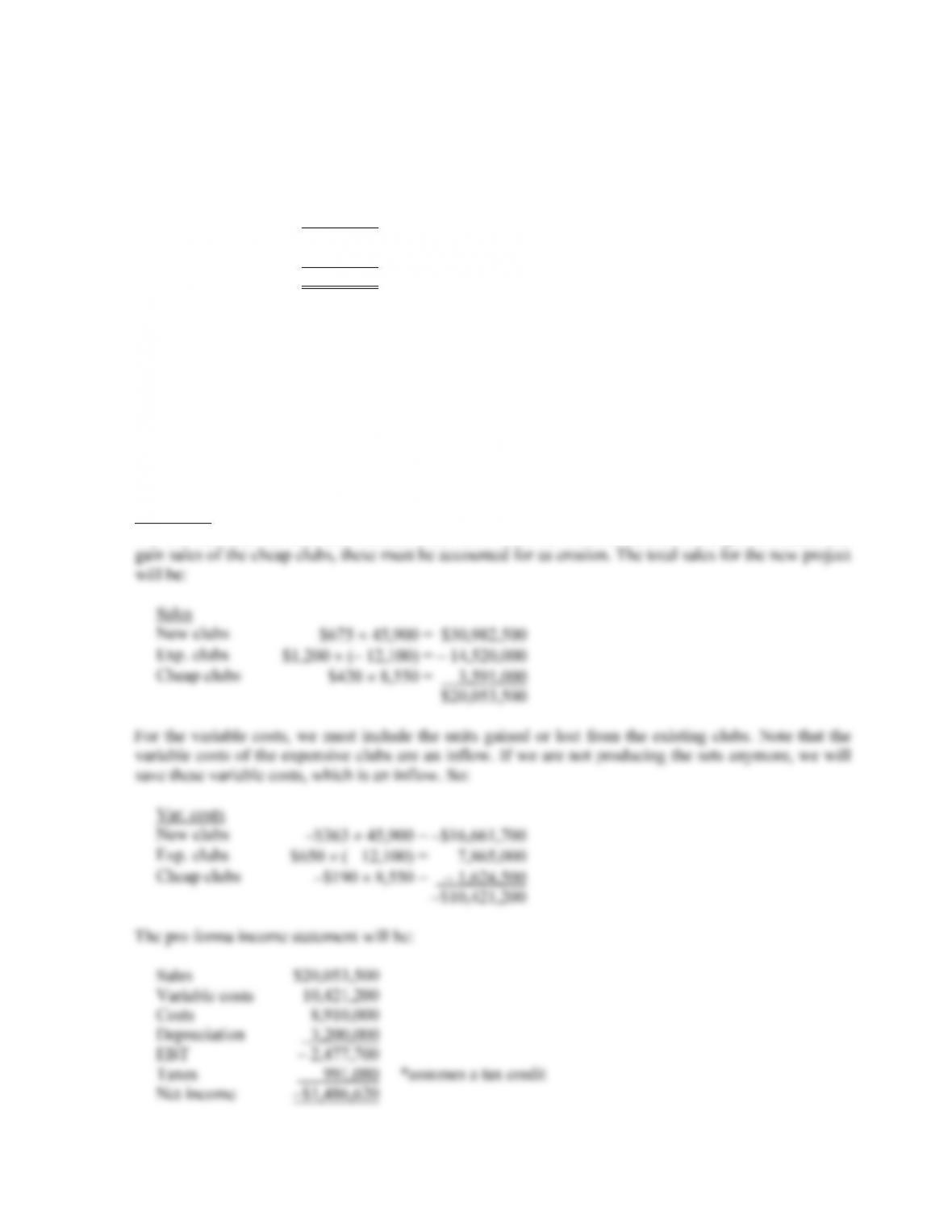B-214 SOLUTIONS
The pro forma income statement will be:
Sales \$38,791,500
Variable costs 12,212,200
Costs 7,290,000
Depreciation 3,200,000
EBT 16,089,300
Taxes 6,435,720
Net income \$9,653,580
Using the bottom up OCF calculation, we get:
OCF = Net income + Depreciation = \$9,653,580 + 3,200,000
OCF = \$12,853,580
And the best-case NPV is:
NPV = –\$22,400,000 – 1,250,000 + \$12,853,580(PVIFA10%,7) + 1,250,000/1.107
NPV = \$39,568,058.39
Worst-case
We will calculate the sales and variable costs first. Since we will lose sales of the expensive clubs and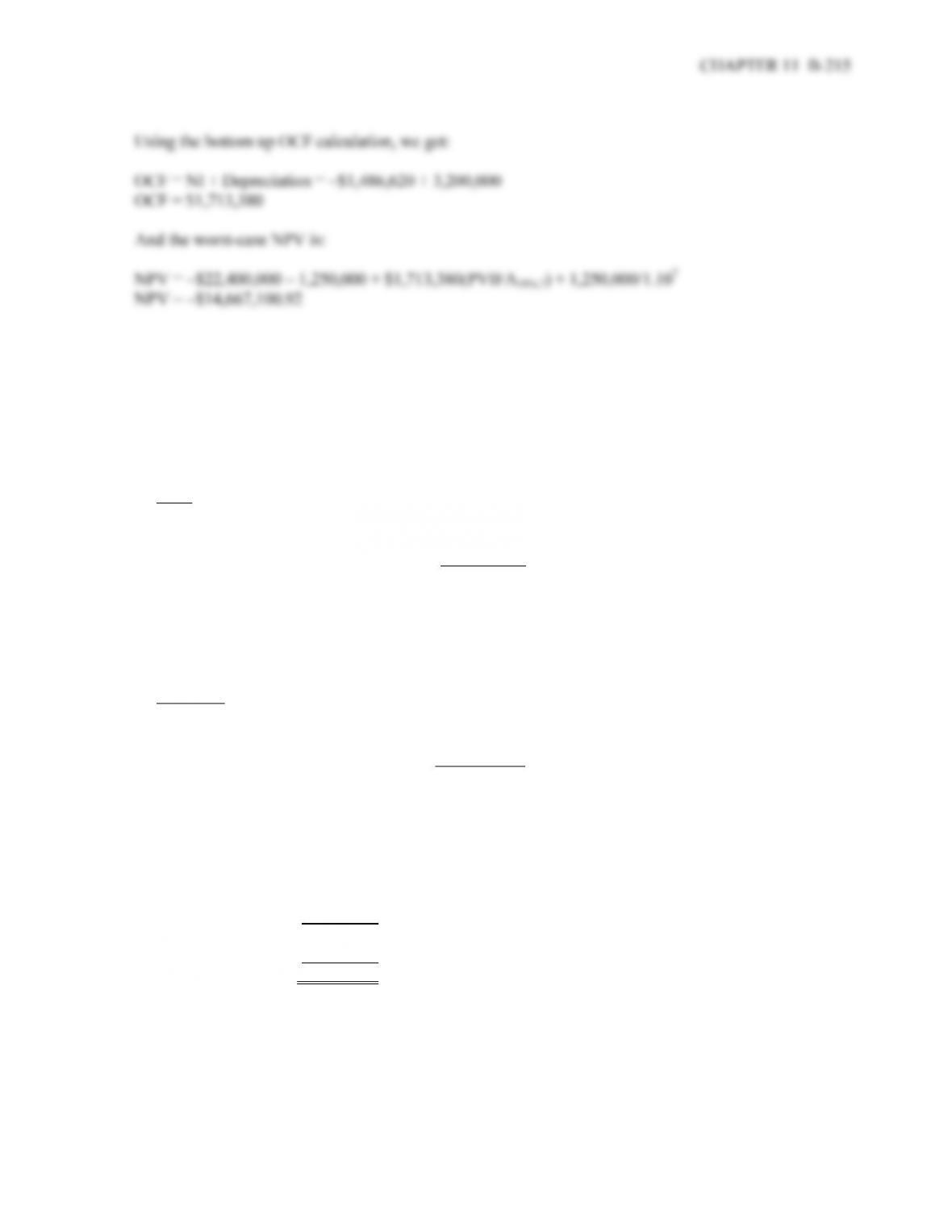22. To calculate the sensitivity of the NPV to changes in the price of the new club, we simply need to
change the price of the new club. We will choose \$800, but the choice is irrelevant as the sensitivity will
be the same no matter what price we choose.
We will calculate the sales and variable costs first. Since we will lose sales of the expensive clubs and
gain sales of the cheap clubs, these must be accounted for as erosion. The total sales for the new project
will be:
Sales
New clubs \$800 51,000 = \$40,800,000
Exp. clubs \$1,200 (–11,000) = –13,200,000
Cheap clubs \$420 9,500 = 3,990,000
\$31,590,000
For the variable costs, we must include the units gained or lost from the existing clubs. Note that the
variable costs of the expensive clubs are an inflow. If we are not producing the sets anymore, we will
save these variable costs, which is an inflow. So:
Var. costs
New clubs –\$330 51,000 = –\$16,830,000
Exp. clubs –\$650 (–11,000) = 7,150,000
Cheap clubs –\$190 9,500 = –1,805,000
–\$11,485,000
The pro forma income statement will be:
Sales \$31,590,000
Variable costs 11,485,000
Costs 8,100,000
Depreciation 3,200,000
EBT 8,805,000
Taxes 3,522,000
Net income \$ 5,283,000
Using the bottom up OCF calculation, we get:
OCF = NI + Depreciation = \$5,283,000 + 3,200,000
OCF = \$8,483,000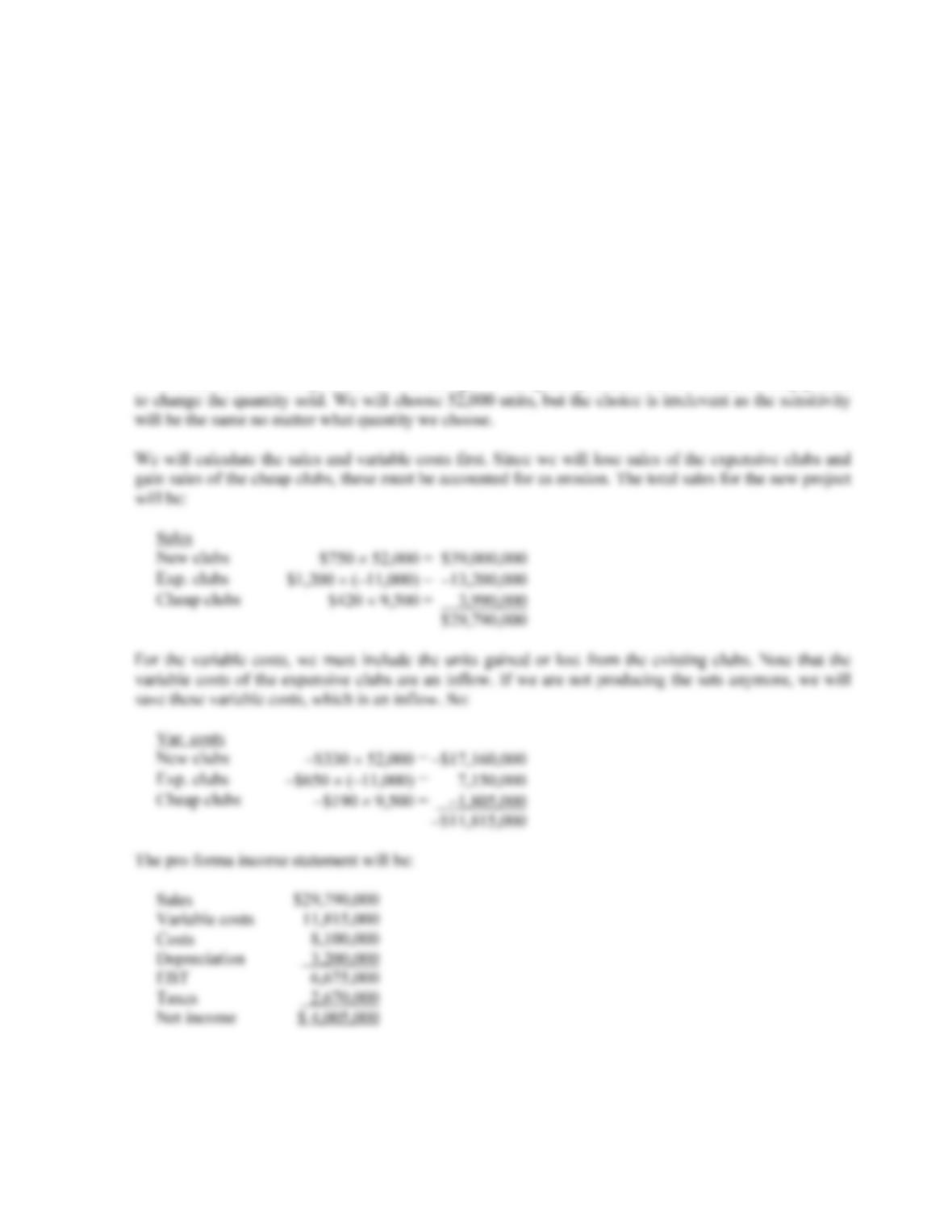B-216 SOLUTIONS
And the NPV is:
NPV = –\$22,400,000 – 1,250,000 + \$8,483,000(PVIFA10%,7) + 1,250,000/1.107
NPV = \$18,290,244.48
So, the sensitivity of the NPV to changes in the price of the new club is:
NPV/P = (\$10,841,563.69 – 18,290,244.48)/(\$750 – 800)
NPV/P = \$148,973.62
For every dollar increase (decrease) in the price of the clubs, the NPV increases (decreases) by
\$148,973.62.
To calculate the sensitivity of the NPV to changes in the quantity sold of the new club, we simply need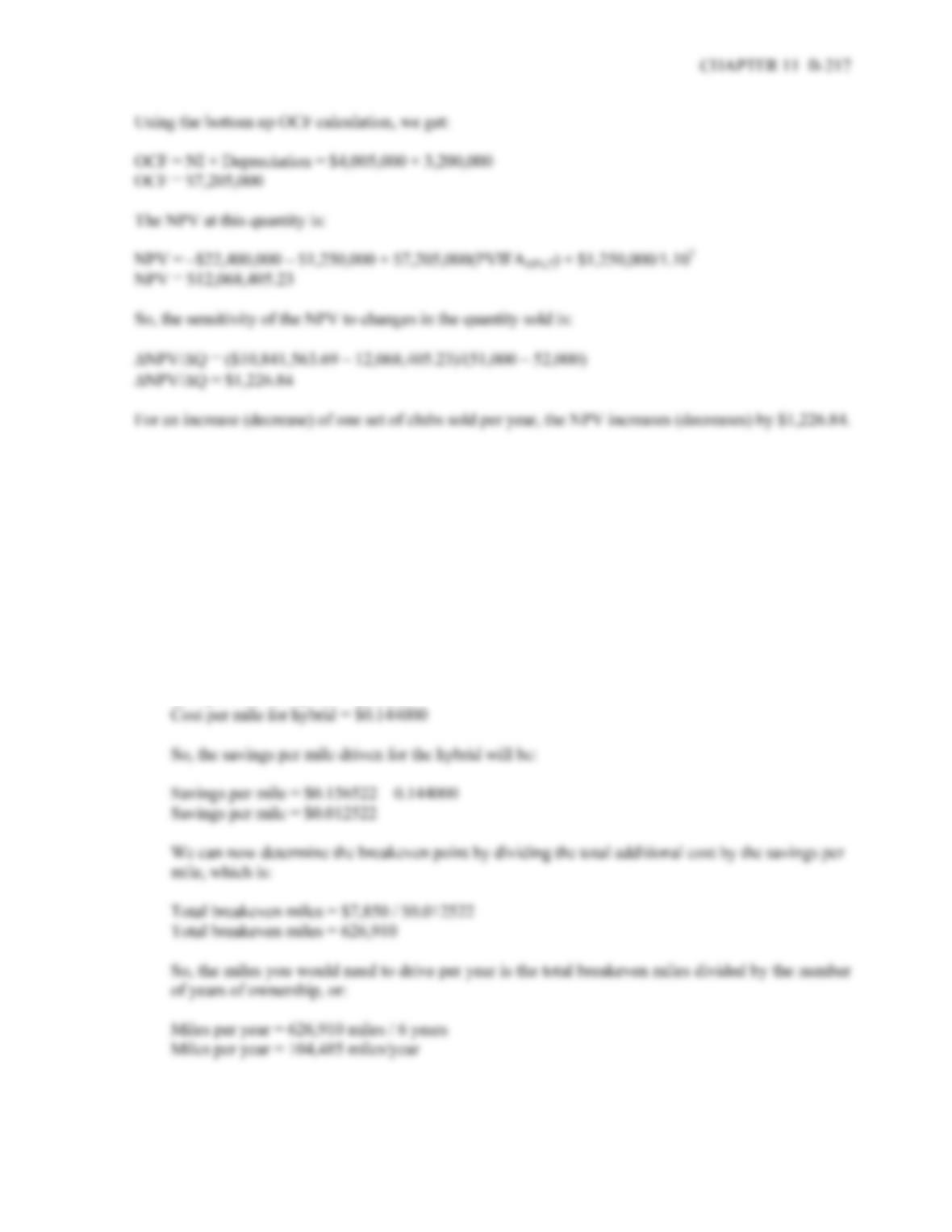23. a. First we need to determine the total additional cost of the hybrid. The hybrid costs more to
purchase and more each year, so the total additional cost is:
Total additional cost = \$5,450 + 6(\$400)